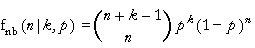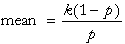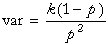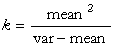# DPL 9 Help

Negative Binomial Distribution

#Negative Binomial Distribution

The Negative Binomial Distribution is the sum of k samples from a Geometric Distribution, and is generally used when a count of some sort is of interest. The distribution represents the number of failures encountered before the kth success, assuming a constant probability of failure on each trial. It has a wide variety of applications, such as the modeling of cosmic rays, learning processes, exposure to diseases, health and accident statistics, psychological data, demand by households for frequently purchased products, observed distributions of consumer expenditure, and as weights (lag distributions) for time series in economics. An example is a model of the frequency of death following repeated exposure to a disease.

The Negative Binomial Distribution can also be used as an approximation for the Poisson distribution when independence of events may not be satisfied.

The Negative Binomial Distribution represents the probability of getting the kth head on the (n+k)th flip of a biased coin. The probability of a head on any flip is p. The parameters of the distribution are k, which must be a positive integer, and p. The distribution is:where k=1,2,..., n=0,1,..., and 0<p<1.

The equations for the mean and variance are:The equations for the parameters are:The mean must be positive and less than the variance. Also the expression for k must produce a positive integer.

Versions: DPL Professional, DPL Enterprise, DPL Portfolio